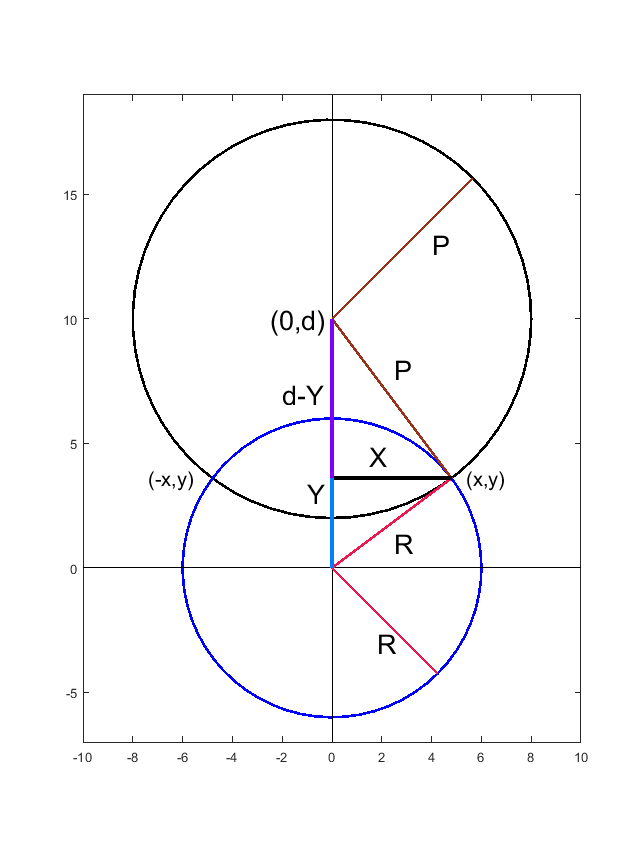# Problem 58852. Intersection(s) of Circles

Do two given circles intersect in Zero, One, or Two points and provide the intersection(s). The Stafford method may provide some guidance and alternate solution method. I will elaborate a more geometric solution utilizing Matlab specific functions, rotation matrix, and translation matrix. Assumption is that Matlab function circcirc is not available.
Given circles [x1,y1,R] and [x2,y2,P] return the intersections [], [x y], or [x y;x y].
The below figure is created based upon d=distance([x1,y1],[x2,y2]), translating (x1,y1) to (0,0), and rotating (x2,y2) to be on the Y-axis. From this manipulation two right triangles are apparent: [X,Y,R] and [X,d-Y,P]. Subtracting and simplifying these triangles leads to Y and two X values after substituting back into R^2=X^+Y^2 equation.
P^2=X^2+(d-Y)^2 and R^2=X^2+Y^2 after subtraction gives R^2-P^2=Y^2-(d-Y)^2 = Y^2-d^2+2dY-Y^2=2dY-d^2 thus
Y=(R^2-P^2+d^2)/(2d) and X=+/- (R^2-Y^2)^.5
The trick is to now un-rotate and translate this solution matrix using t=atan2(dx,dy), [cos(t) -sin(t);sin(t) cos(t)] and [x1 y1]Diagram showing a normalization of posed problem where (x1,y1) is placed at origin and (x2,y2) is placed on Y-axis at distance d. Final (x,y) values will require rotation and shifting.

### Solution Stats

90.91% Correct | 9.09% Incorrect
Last Solution submitted on Oct 01, 2023

### Community Treasure Hunt

Find the treasures in MATLAB Central and discover how the community can help you!

Start Hunting!